# 在Unity URP管线下 基于Forward路径 快速实现屏幕空间反射(SSR)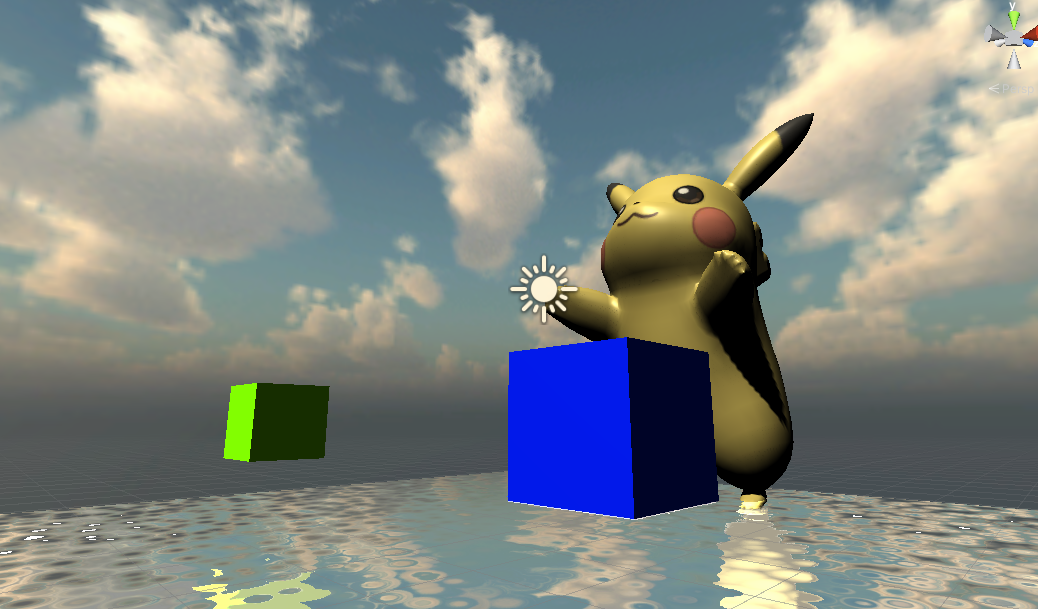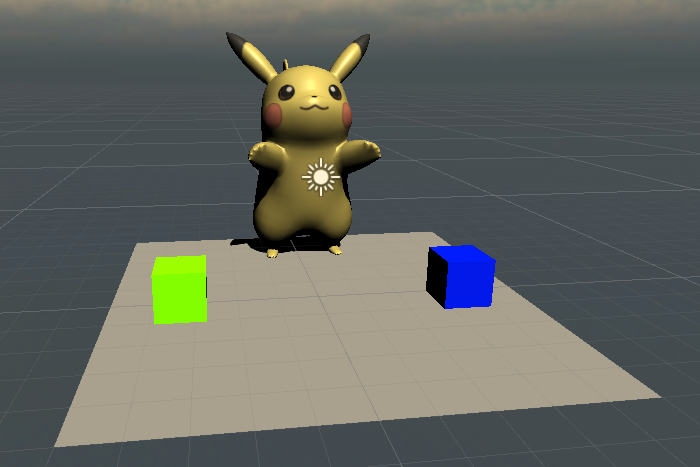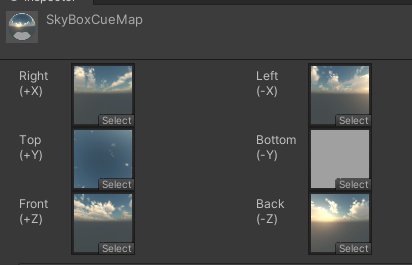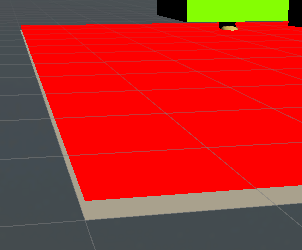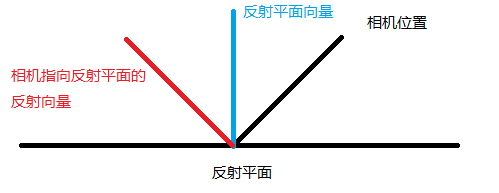1.根据相机方向和反射物件的位置，计算出视野到物体的向量，之后根据物体平面方向向量计算出反射向量
2.以视野方向跟物体相交的位置作为反射起点（反射像素将要填充的位置），以反射向量为方向进行步进， 在每次步进时投影回屏幕空间，进行屏幕空间深度碰撞检测
3.当发现屏幕空间深度和当前步进的检测点相交，或者接近 则视为碰撞成功，返回当前相交点的屏幕空间坐标(UV) 对 CameraOpaqueTexture进行采样像素回贴到反射起点， 否则继续步进，为了防止无限步进导致性能下降，设定一个最远步进距离，超过时，返回天空盒

 12345678910111213141516171819202122232425 v2f vert (appdata v)             {                 v2f o;                 o.vertex = TransformObjectToHClip(v.vertex);                 o.uv = v.uv;                 o.positionWS = TransformObjectToWorld(v.vertex).xyz;                 o.positionOS = v.vertex.xyzw;                 float4 screenPos = TransformObjectToHClip(v.vertex);                 screenPos.xyz /= screenPos.w;                 screenPos.xy = screenPos.xy * 0.5 + 0.5;                 o.positionCS = screenPos; #if UNITY_UV_STARTS_AT_TOP                 o.positionCS.y = 1 - o.positionCS.y; #endif                 float zFar = _ProjectionParams.z;                 float4 vsRay = float4(float3(o.positionCS.xy * 2.0 - 1.0, 1) * zFar, zFar);                 vsRay = mul(unity_CameraInvProjection, vsRay);                 o.vsRay = vsRay;                 return o;             }

 12 float3 viewDirWS = normalize(positionWs.xyz -  WorldSpaceCameraPos.xyz) float3 viewDirVS = mul(UNITY_MATRIX_V, float4(viewDirWS,0))

 1 float4 vsRay = float4(float3(o.positionCS.xy * 2.0 - 1.0, 1) * zFar, zFar);

 12345678910111213141516171819202122232425262728 float4 frag (v2f i) : SV_Target             {                 float4 screenPos = i.positionCS;                 float depth = SAMPLE_DEPTH_TEXTURE(_CameraDepthTexture, sampler_CameraDepthTexture, screenPos.xy);                 depth = Linear01Depth(depth, _ZBufferParams);                                 float3 wsNormal = normalize(float3(0, 1, 0));    //世界坐标系下的法线                 float3 vsNormal = (TransformWorldToViewDir(wsNormal));    //将转换到view space                 float3 vsRayOrigin = (i.vsRay) * depth;                 float3 reflectionDir = normalize(reflect(vsRayOrigin, vsNormal));                 float2 hitUV = 0;                 float3 col = 0;                 if (rayMarching(vsRayOrigin, reflectionDir, hitUV))                 {                     float3 hitCol = SAMPLE_TEXTURE2D(_CameraOpaqueTexture, sampler_CameraOpaqueTexture, hitUV).xyz;                     col += hitCol;                 }                 else {                     float3 viewPosToWorld = normalize(i.positionWS.xyz - _WorldSpaceCameraPos.xyz);                     float3 reflectDir = reflect(viewPosToWorld, wsNormal);                     col = SAMPLE_TEXTURECUBE(_SkyBoxCubeMap, sampler_SkyBoxCubeMap, reflectDir);                 }                 return float4(col, 1);             }

 1 float3 vsRayOrigin = (i.vsRay) * depth;

 12345678910111213141516171819202122232425262728293031323334353637383940 float compareWithDepth(float3 vpos)             {                 float2 uv = ViewPosToCS(vpos);                 float depth = SAMPLE_TEXTURE2D(_CameraDepthTexture, sampler_CameraDepthTexture, uv);                 depth = LinearEyeDepth(depth, _ZBufferParams);                 int isInside = uv.x > 0 && uv.x < 1 && uv.y > 0 && uv.y < 1;                 return lerp(0, abs(vpos.z + depth), isInside);             }             bool rayMarching(float3 o, float3 r, out float2 hitUV)             {                 float3 start = o;                 float3 end = o;                 float stepSize = 0.15;                 float thinkness = 0.1;                 float triveled = 0;                 int max_marching = 256;                 float max_distance = 500;                 UNITY_LOOP                 for (int i = 1; i <= max_marching; ++i)                 {                     end += r * stepSize;                     triveled += stepSize;                     if (triveled > max_distance)                     return false;                     float collied = compareWithDepth(end);                     if (collied > 0)                     {                         if (collied < thinkness)                         {                             hitUV = ViewPosToCS(end);                             return true;                         }                     }                 }                 return false;             }

LinearEyeDepth(depth, _ZBufferParams); 计算的结果是距离当前相机的距离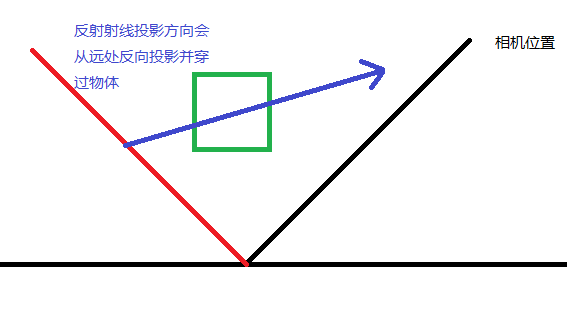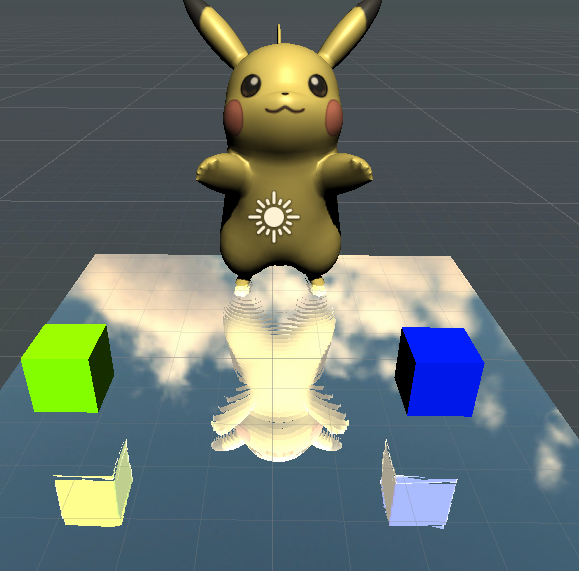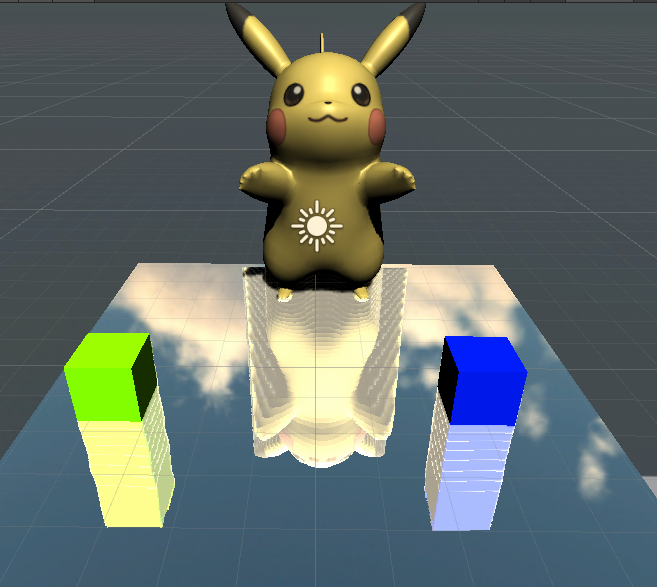123456789101112131415161718 ... float stepSize = 0.5; //这里可能将步长加大 ...                     float collied = compareWithDepth(end);                     if (collied < 0)                     {                         if (abs(collied) < thinkness)                         {                             hitUV = ViewPosToCS(end);                             return true;                         }                         //回到当前起点                         end -= r * stepSize;                         triveled -= stepSize;                         //步进减半                         stepSize *= 0.5;                     }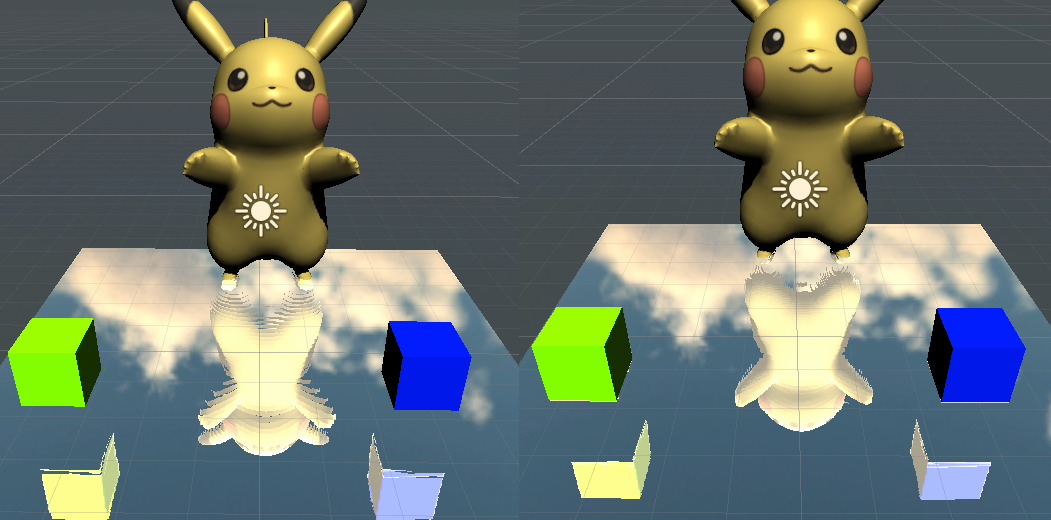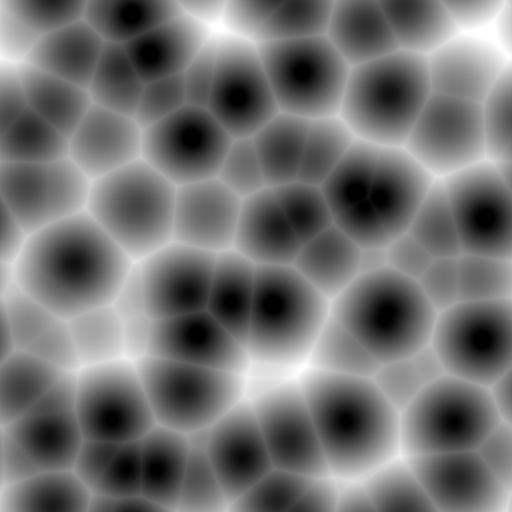1234 ... float2 noiseTex = (SAMPLE_TEXTURE2D(_Noise, sampler_Noise, (i.uv * 5) + _Time.x).xy * 2 - 1) * 0.1; float3 wsNormal = normalize(float3(noiseTex.x, 1, noiseTex.y));    //世界坐标系下的法线 ...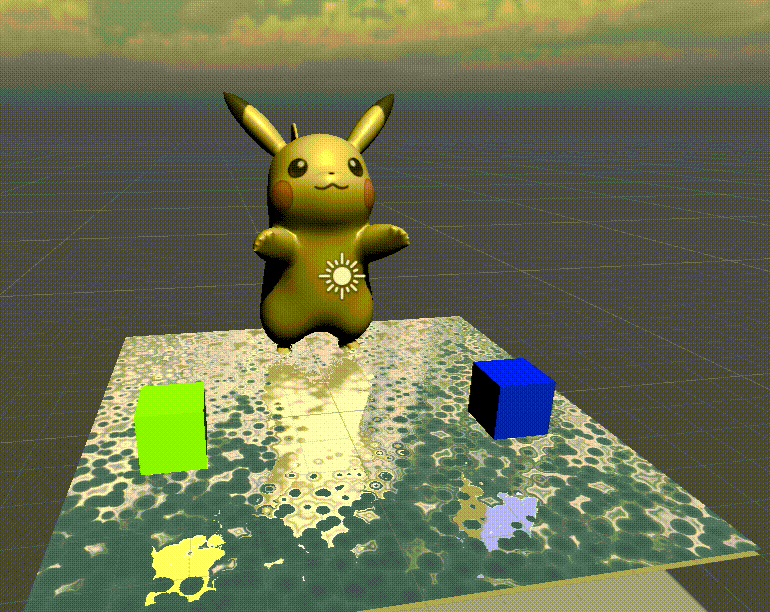This site uses Akismet to reduce spam. Learn how your comment data is processed.